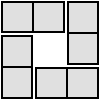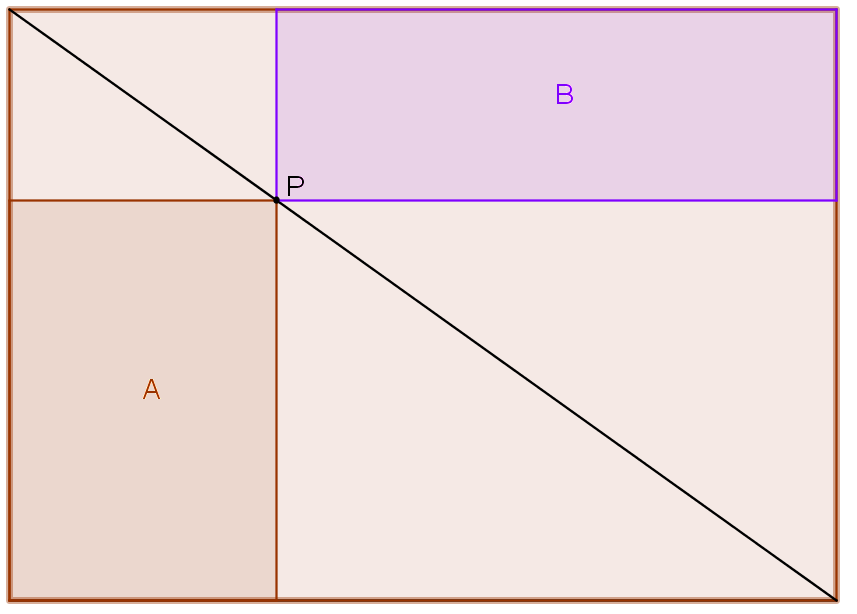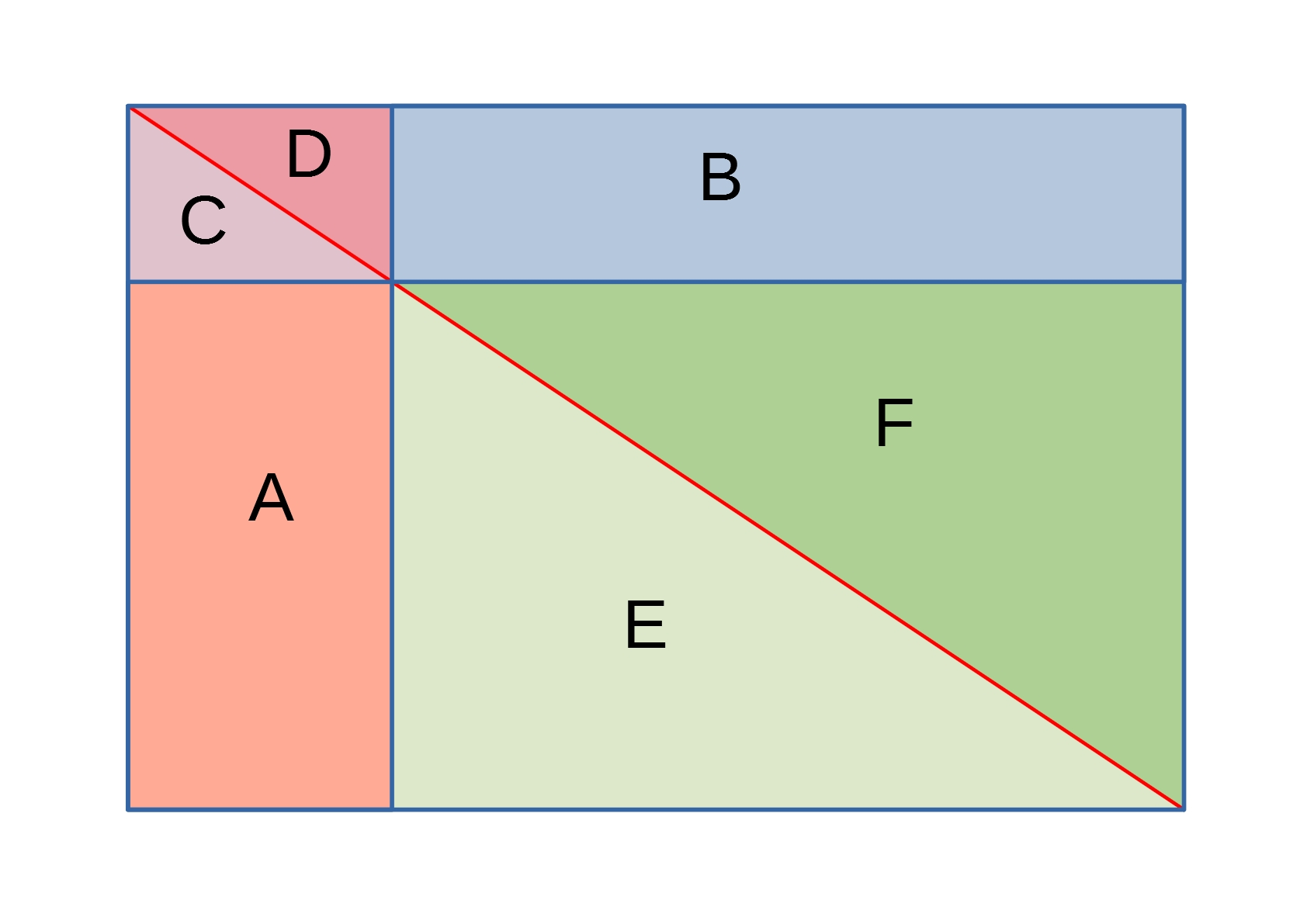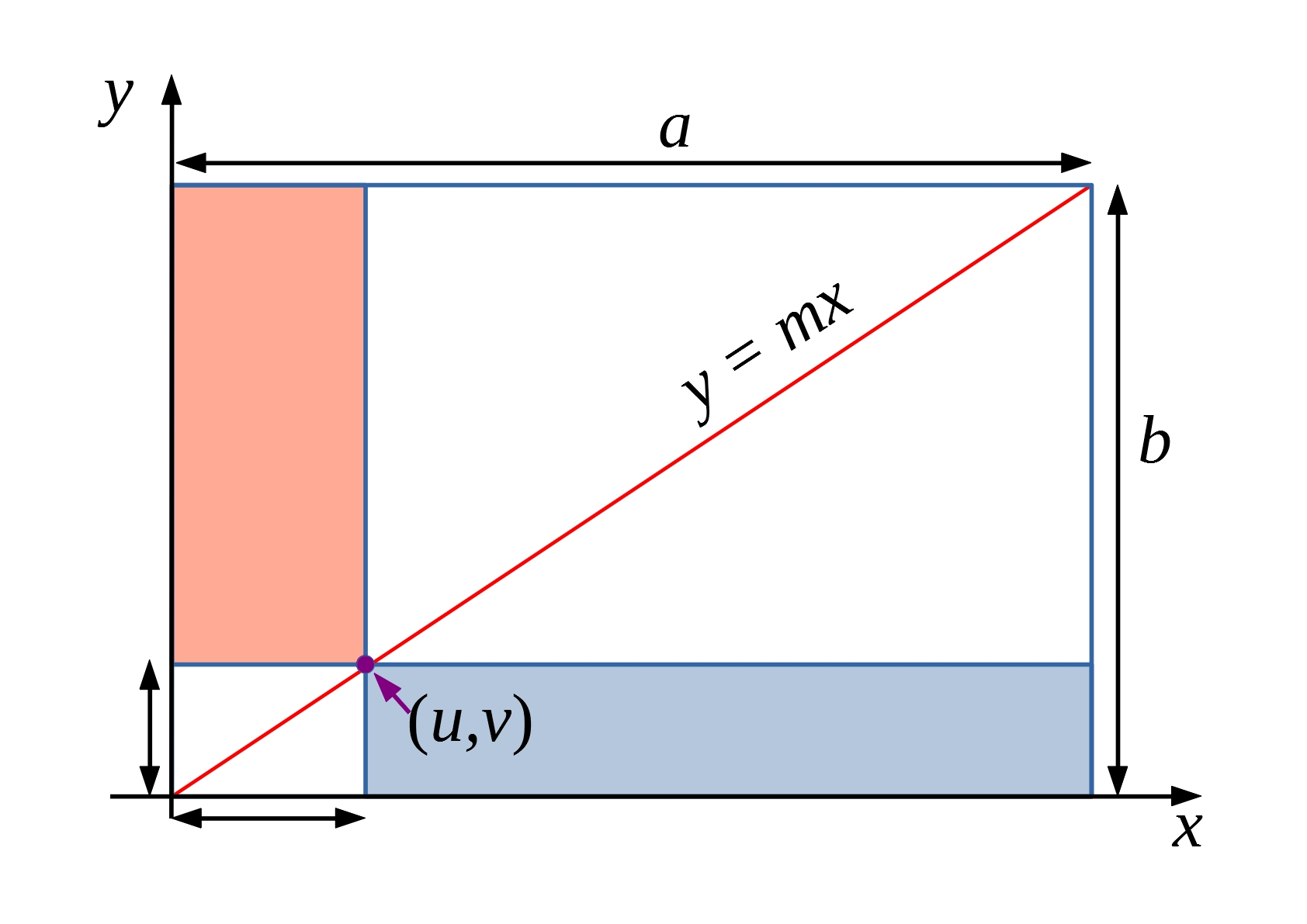#### You may also like### Teddy Town

There are nine teddies in Teddy Town - three red, three blue and three yellow. There are also nine houses, three of each colour. Can you put them on the map of Teddy Town according to the rules?### 4 Dom

Use these four dominoes to make a square that has the same number of dots on each side.### Just Rolling Round

P is a point on the circumference of a circle radius r which rolls, without slipping, inside a circle of radius 2r. What is the locus of P?

# Sliding Rectangles

##### Age 11 to 16Challenge LevelThe picture to the right shows a rectangle with a diagonal drawn on it.

An arbitrary point, $P$, has been placed on the diagonal.

The two shaded rectangles, $A$ and $B$, have a common vertex at $P$.

Which of these two rectangles has the greater area?

Below is an interactive version of the picture above. You may find it helpful to click and drag point $P$, and see how the two rectangles $A$ and $B$ change:

Can you use the diagrams below to justify your results in two different ways?